In May of 1999, Ian Stewart issued the article “A Puzzle for Pirates” in that month's edition of Scientific American (pp.98-99). This mathematical game then went on to become a worldwide hit, especially for puzzlers who like a good challenge. The rules of the pirate game are described below:

Five rational pirates have found 100 gold coins on an island and need to decide how to distribute them. They are a democratic bunch and they ask the fiercest pirate to propose a plan of distribution. Then, all of the living pirates (including the proposer) vote on whether to accept the distribution plan. If the majority (50% or more) accepts that proposal, then coins are dispersed and the game ends. Otherwise, the proposer is thrown overboard and killed, then the procedure is repeated with the next fiercest pirate until a proposal is accepted or only one pirate is left. If there is a tie vote, then the proposer (say, the fiercest pirate) has the casting vote.

At first glance, the proposer may offer the most favorable terms to all voters to pass the proposal, but this is not the case. The pirates are not only rational but also greedy and want to maximize their personal gain. Due to the game's rules and how the order of pirate fierceness is known to all in advance, these rational pirates prioritize surviving first, then maximizing gold coins for themselves. They would love to kill off as many pirates as possible to make more coins for themselves through future distribution proposals. This logic may become surprisingly complicated due to the changes in the numbers of pirates and coins.

Suppose N is the number of pirates, and G is the number of gold coins. We number the pirates in order from meekest to fiercest, the greater the number, the fiercer the pirate. So P1 is the least fierce pirate, and PN is the fiercest pirate. Due to there being too many branches from the beginning, we can think of the problem backward from the end, that is, starting from the game results to reverse the entire distribution process.

If there are only two pirates (N=2), then obviously the fierce pirate is going to propose G coins for himself, and none for the meek pirate (P1=0, P2=G). Any proposal will pass no matter what it is for this case, so the fierce pirate would take all the coins while the meek pirate has no choice but to accept it. The fierce pirate doesn’t need to please meek pirate at all!

If there are three pirates (N=3), and all of them are aware of an inevitable positive result, then the fiercest pirate P3 is going to make a proposal pass to guarantee his own survival first, so he needs at least one vote from P1 or P2. Due to P2 having the possibility of getting G coins, so he can’t be bribed with gold coins; P1 knows he is going to get 0 coins if the game goes onto the next round, and P3 knows what P1 is thinking, so P3 can offer the least amount of coins, e.g., just 1 coin to buy the vote of P1, and P1 also would love to support P3 accordingly as he can get a better result: survival plus coins. So, the final proposal by P3 is P1=1, P2=0, P3=G-1, where G>1.

From the two trial calculations above, we can conclude the following decision-making patterns or rules for a player of the pirate game. The rules apply to all pirates and it is the rationality of a pirate, that is the pirate game recursive solving algorithm (click here to download the precompiled code).

1. For any N, G, both N and G must be greater than zero for game, to avoid the possibility of unlimited bribing, we usually define them as both integer and 2*G < N, but this is not required.
2. If the number of pirates N is 2, then proposal is P1=0, P2=G.
3. Otherwise, count the proposer’s vote out and let the rest of the pirates (N-1) to distribute G gold coins. For its trial result proposal, count the number of votes supported:
• The fiercest pirate whose proposal is impossible to pass in the next round. Because this pirate is going to die in the next round, he is the inevitable supporter of the current proposal to avoid making a proposal and being killed in the next round.
• The pirates who are going to receive 0 coins in the next round. Try to buy this kind of pirate as a supporter with the least coins (e.g., 1 coin) from total G coins. For those pirates who received coins in the trial calculation, take their coins away!
4. Check if the current proposal is going to pass the vote and return that flag.
• If the number of votes supported plus 1 vote of the current proposer is equal to or greater than the N/2, then the proposal is passed. So the current proposer takes all the remaining gold coins after buying supporters in 3.b and make all those pirates going to die in the next round alive because they avoid the further distribution process.
• If the current proposal cannot be passed, then throw the current proposer overboard, marking PN as a must-die pirate.

Please note step #3, we're calling the pirate game-solving algorithm recursively, so it would reach the game-over condition. The following proposal plan table is generated by upper algorithm implementation by SAS for pirate games with N<=20 and G=100. You can see the best solution is, the fiercest pirate chooses the pirates with the same parity as him in the list ordered by fierce as his supporter. E.g., P3 would choose P1 as his supporter for a N=3 pirate game, and P5 would choose P3, P1 as his supporter for a N=5 game.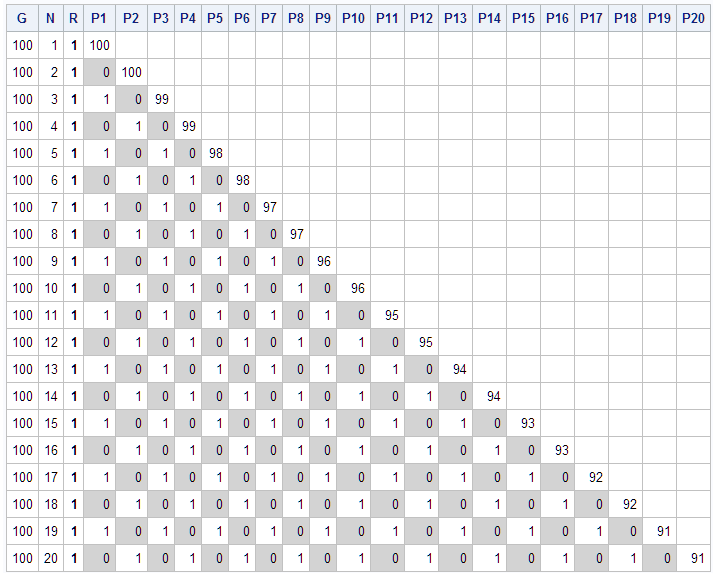Will this pattern continue for any N pirate games with constant G gold coins? The answer is no because we have limited gold coins (G) to buy supporters. Anyway, it is true for N<= 2*G game, e.g., The P200 can take 1 coin and buy 99 supporters with another 99 coins, the detailed proposal plan for N=200 pirate game is show as below: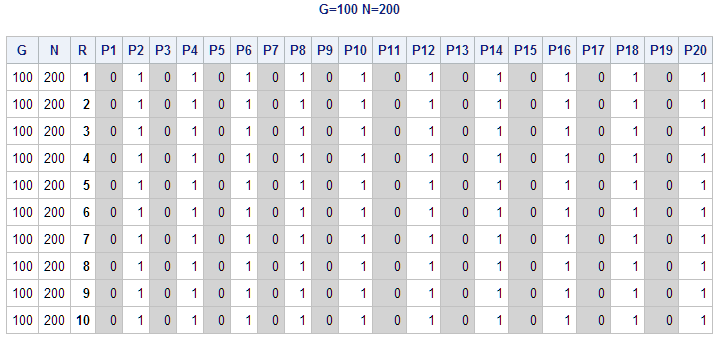Interesting things happen while the number of pirates N exceed the 2*G. e.g., P201 and P202 pirates still can survive if he is taking no gold coins for himself and offering 1 gold coin each to 100 pirates with the same parity in the list ordered by fierceness. The detailed proposal for N=201, N=202 cases is show as below: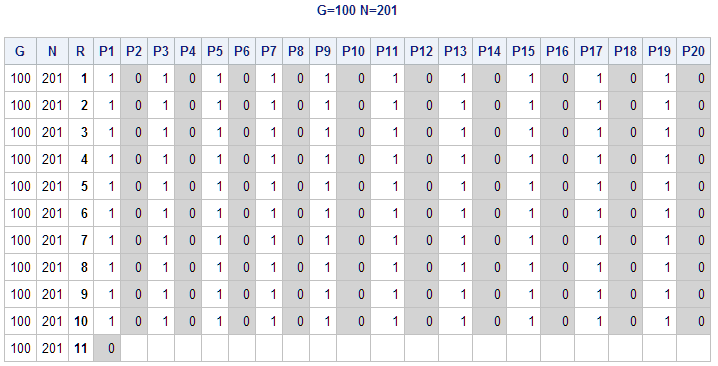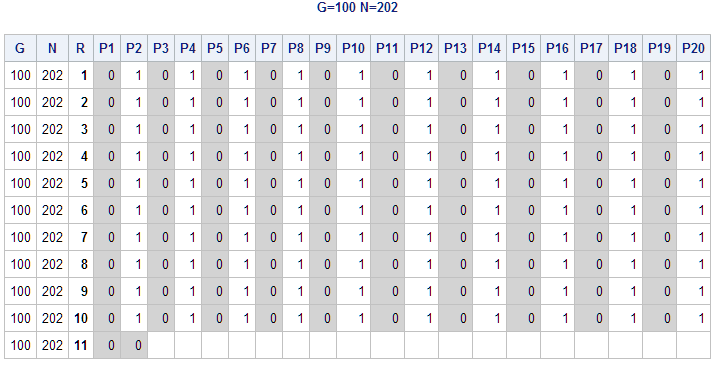Unfortunately, P203 has no coins to buy supporters anymore in the pirate game (G=100, N=203), so P201 and P202 in this round would love to throw P203 overboard to earn more coins through future distribution proposals. In other words, P203 must die for this case (We use -1 to indicate death in detailed proposal).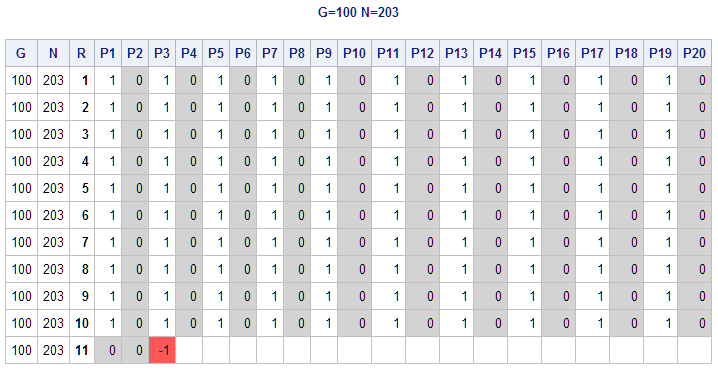P204 is a lucky guy who can survive. Since P203 must die in the next round, P203 is going to support any proposal made by P204 in the current round. P204 will apply the same strategy as P202 used in the N=202 pirate game above, so P204 takes no coins, and he gets support from P203 in this current round. So, P201, P202, P203 and P204 all survive with no coins. The detailed proposal is shown as below: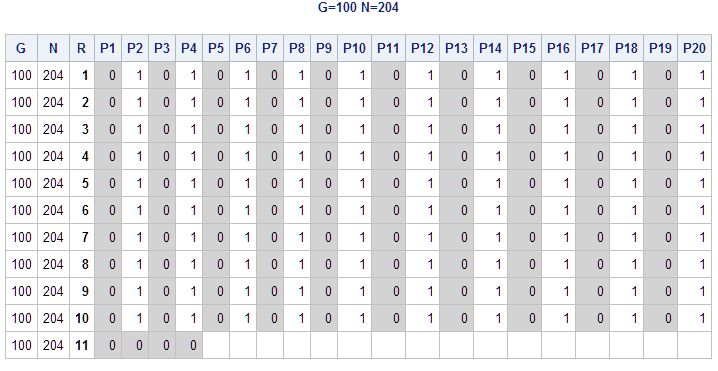Accordingly, P205, P206 and P207 are all unlucky and doomed to die in their round, just like P203 in pirate game (G=100, N=203). The key reason is that they cannot get sufficient votes to make their proposals pass. The four rational pirates (P201 to P204) know they can survive and would love to throw these three pirates (P205 to P207) overboard to earn more coins through future distribution proposals. The detailed proposal for the game (G=100, N=207) is shown below: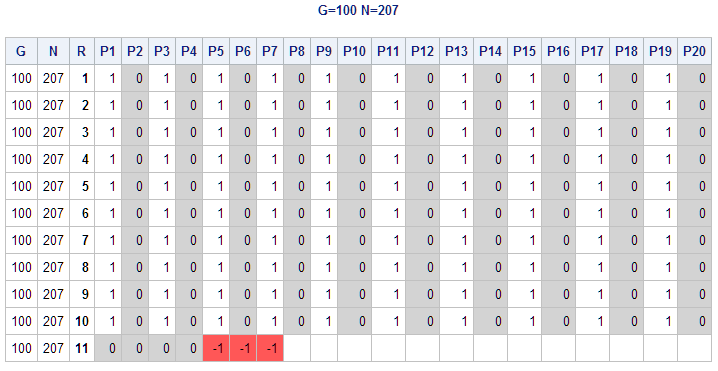Just as lucky as P204 in pirate game (G=100, N=204), P208 is also lucky enough to make a proposal pass because he can get sufficient support from P205, P206 and P207 who are doomed to die. P205, P206 and P207 must support P208 to avoid making a proposal and getting killed in the next round. The detailed proposal for the game (G=100, N=208) is shown below: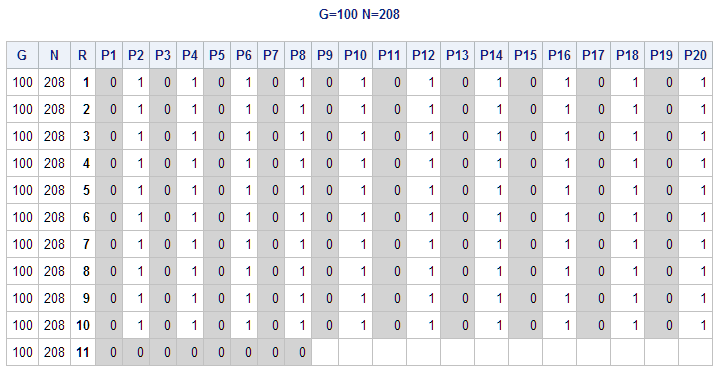In general, suppose the number of the pirate I-th (I<= N) is PI in a pirate game (N, G), we have the following conclusion based on the recursive solving algorithm result:

1. When I<=2*G, all pirates will survive, and they also have 50% possibility to get 1 or more gold coins.
2. When I >2*G and I=N, the pirate PI (=PN, proposer) is going to survive only when (N - 2*G is the power of 2, otherwise they will die.
3. When I>2*G and I<N, all pirates with number I <= (2*G + M) will survive but will receive no coins, all pirates whose number I>(2*G+M) will die, where M is the highest power of 2 that does not exceed N - 2*G. Please note that the survival for the PI is a possibility related to N value, those PI will survive again If N exceed 2*G + 2*M.

e.g., G=100, N=(205, 206, 207), N-2*G=(5, 6, 7), the M=4 (2^2<=4), so 2*G+M=204, so P201, P202, P203 and P204 will survive in pirate games N=(205, 206, 207). P205 must die in pirate game N=(205, 206, 207), and P206 must die in pirate game N=(206, 207), and P207 must die in pirate game N=207. But P205, P206, P207 will survive again if N is equal to or greater than 208.

In fact, there is no unique solution to distribute gold coins if a pirate already guarantees his survival. This means that the fiercest pirate has some freedom of selection when I>=2G+2, but the simplest solution is to select those pirates with the same parity by order of fierceness.

From the proposer’s perspective (I=N), if he attends a pirate game with different N, then his personal interest is different. For a game with a number of pirates that is less than or equal to 2*G, the proposer will always survive with coins. For games with greater than 2*G pirates, most proposers can’t survive except whose number minus 2*G happens to be an integer power of 2. We can visualize the solution for pirate game(N=500, G=100) with SAS as seen below.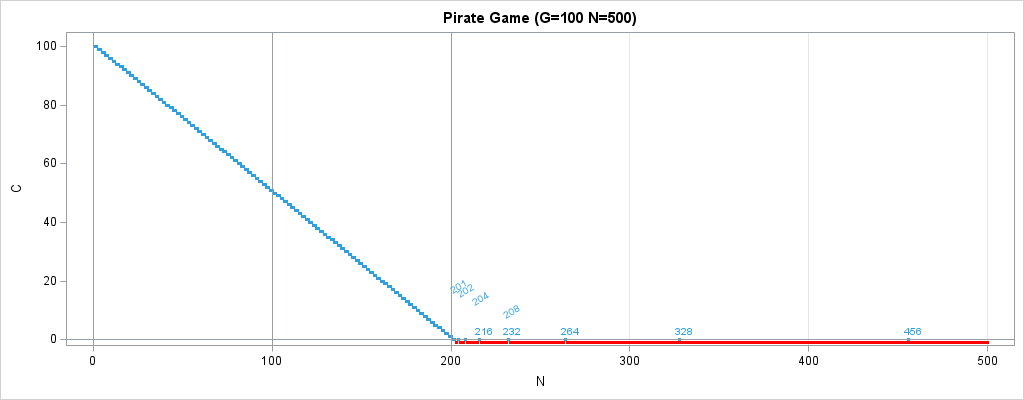Accordingly, we also can calculate the possibility of survival of a pirate game with N pirates (N<=500) and constant G=100. The survival curve below indicates how likely a pirate (proposer) is to survive if he participates in a game with N pirates. As expected, the curve is a non-linear line.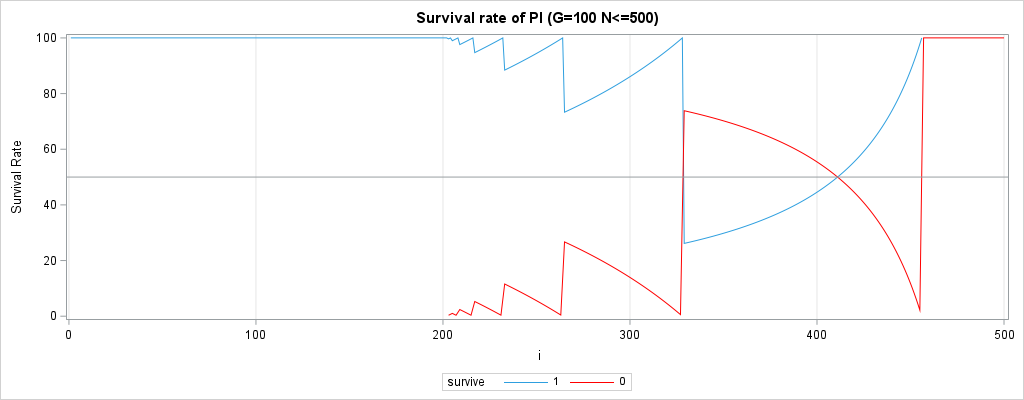For the least fierce pirate, he has no chance to distribute gold coins, but he has most chances to vote on proposals. In other words, he can survive longer than other pirates. For the fiercest pirate, the survival rate appears as a cliff-like fall at some points for the pirate whose number PI is greater than 2*G. The survival curve below indicates how likely a pirate PI is to survive if he participates in a game with N pirates (N<=500).

e.g., For a game with 500 pirates, the first 44 pirates are doomed to be thrown overboard (P457 to P500), so the blue survival line falls to 0 at P456 (2*G+2^Y=2*100+2^8=456), accordingly the death rate(red) is suddenly increased to 100%.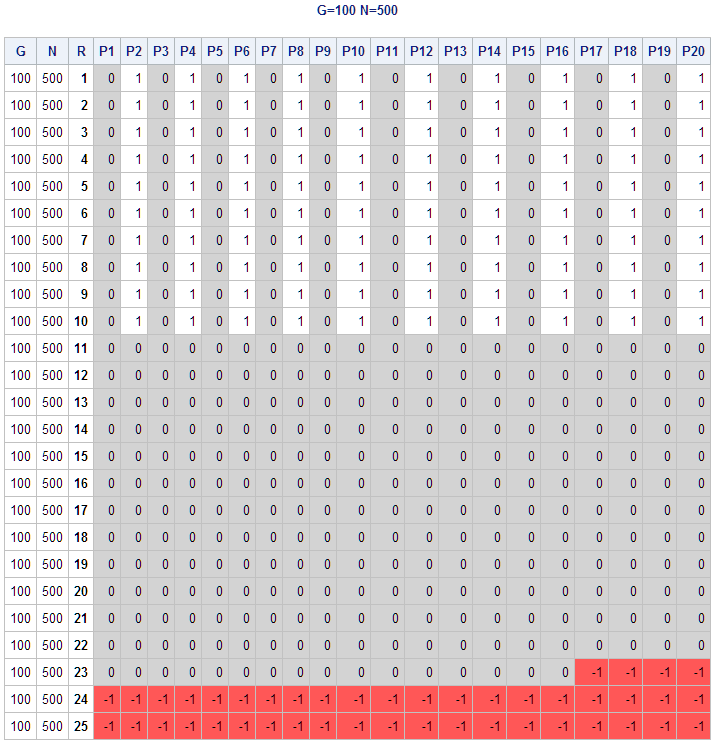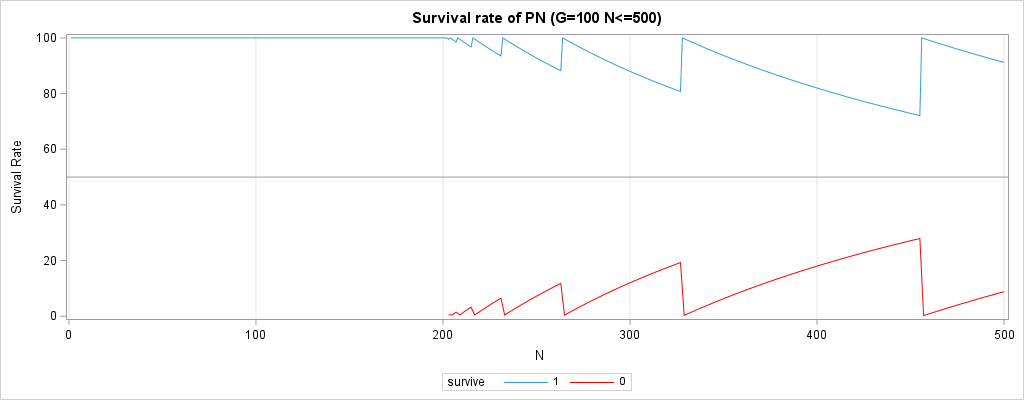Summary

We have figured out how to solve the pirate game with a recursive solving algorithm in SAS, and how to analyze and visualize the law behind the complex logic of the pirate game. Now the next time you join a pirate game, you will know your destiny ahead of time and reap the benefits while avoiding getting thrown overboard.

Solve "A Puzzle for Pirates" with SAS was published on SAS Users.In this post, we tackle the problem of calculating the length of overlap of two date/time intervals. For example, we might have two events — each lasting several days — and need to calculate the number of shared days between both events. In other words, we need to calculate the length of the events’ overlap.

Such tasks are typical in clinical trials programming, health (and non-health) insurance claim processing, project management, planning and scheduling, etc.

The length of the overlap can be measured not only in days, but also in any other units of the date/time dimension: hours, minutes, seconds, weeks, months, years and so on. Moreover, the date/time application is just one special case of a broader task of calculating the overlap length of two integer intervals. An integer interval [x .. y] is a set of all consecutive integer numbers beginning with x and ending with y (boundaries included and x ≤ y).

Instead of suggesting a single “best” way of solving this problem, I offer three different strategies. This allows you to compare and weigh their pros and cons and decide for yourself which approach is most suitable for your circumstances. All three solutions presented in this blog post are applicable to the date/time use case, as well as its superset of integer intervals’ overlap length calculation.

## Problem description

Suppose we have two events A [A1 .. A2] and B [B1 .. B2]. Event A lasts from date A1 until date A2 (A1 ≤ A2), and event B lasts from date B1 until date B2 (B1 ≤ B2).  Both starting and ending dates are included in the corresponding event intervals.

We need to calculate the overlap between these two events, defined as the number of days that belong to both events A and B.

## Overlapping intervals: sample data

Before solving the problem, let’s create sample data to which we are going to apply our possible solutions.

The following data step creates data set EVENTS representing such a data sample:

```data EVENTS; input A1 A2 B1 B2; format A1 A2 B1 B2 date9.; informat A1 A2 B1 B2 mmddyy10.; lines; 01/02/2022 01/05/2022 01/06/2022 01/10/2022 01/22/2022 01/30/2022 01/16/2022 01/18/2022 01/02/2022 01/05/2022 01/03/2022 01/10/2022 01/02/2022 01/05/2022 01/03/2022 01/04/2022 01/10/2022 01/15/2022 01/06/2022 01/14/2022 01/01/2022 01/05/2022 01/05/2022 01/09/2022 01/07/2022 01/13/2022 01/10/2022 01/13/2022 ;```

Now let’s go over the following three distinct possible solutions, each with its own merit and downside. At the end, you decide which solution you like the most.

## Solution 1: Brute force

In general, brute force algorithms solve problems by exhaustive iteration through all possible choices until a solution is found. It rarely results in a clever, efficient solution. However, it is a straightforward, valid and important algorithm design strategy that is applicable to a wide variety of problems.

For integer intervals overlap calculation, a brute force approach would involve the following steps:

1. Determine the earliest date in the definitions of our two intervals
2. Determine the latest date in the definitions of our two intervals
3. Create an iterative loop with index variable ranging from the earliest to the latest date
4. Within this loop, increment a counter by one if index variable falls within both intervals
5. At the end of this loop, the counter will equal the desired value for the overlap.

Here is the code implementation of the described above algorithm:

```data RESULTS; set EVENTS; OVERLAP = 0; do i=min(A1,B1) to max(A2,B2); if (A1<=i<=A2) and (B1<=i<=B2) then OVERLAP + 1; end; run;```

As you can see, the code implementation of the brute force solution is quite simple. However, it is hardly efficient as it involves extensive looping.

## Solution 2: Exhaustive logic

Exhaustive logic algorithms solve problems by splitting them into several smaller problems (logical units or classes). This way, instead of working on a problem in its entirety, you can work separately on smaller and simpler problems that are easier to comprehend, digest and solve.

Here is an illustration of various arrangements of integer intervals representing separate logical units (numbered 1...5) when calculating the intervals overlaps: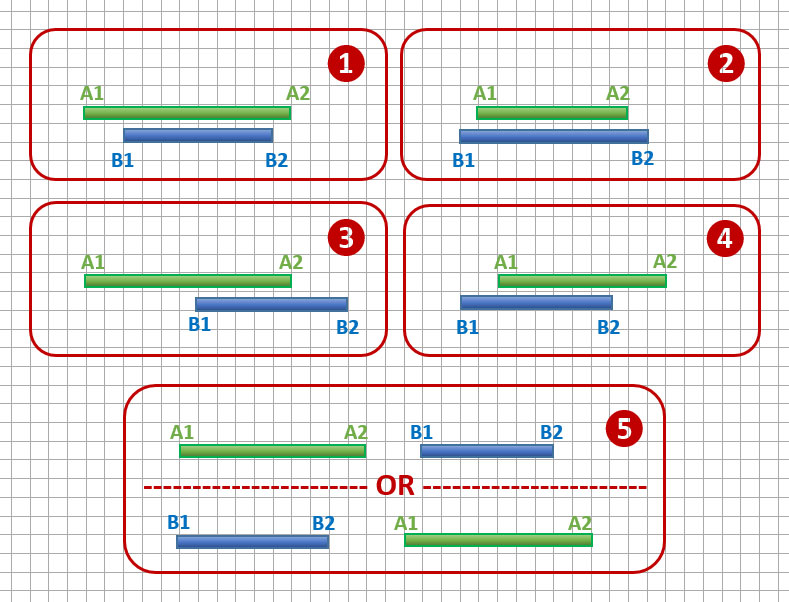The following SAS code demonstrates an implementation of the exhaustive logic algorithm (each IF-THEN statement corresponds to the numbered logical unit in the above figure):

```data RESULTS; set EVENTS; if A1<=B1 and A2>=B2 then OVERLAP = B2 - B1 + 1; else if A1>=B1 and A2<=B2 then OVERLAP = A2 - A1 + 1; else if A1<=B1<=A2 and B2>A2 then OVERLAP = A2 - B1 + 1; else if B1<=A1<=B2 and A2>B2 then OVERLAP = B2 - A1 + 1; else if B1>A2 or A1>B2 then OVERLAP = 0; run;```

Notice that we need to add 1 to each of the two days difference. That is because the number of days spanned is always 1 less than the number of days contained in an interval. Here is an illustration: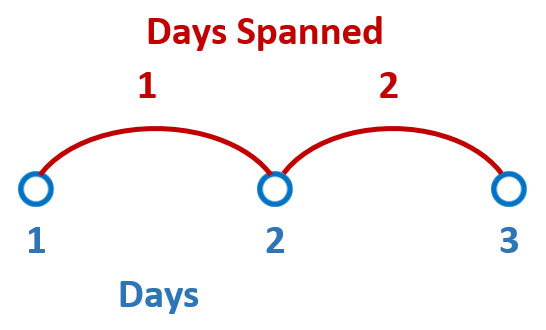## Solution 3: Holistic Math

Finally, a holistic math method looks at the problem in its entirety and condenses it to a comprehensive mathematical formula. While it is usually not the most obvious approach, and often requires some brain stretching and internalization, the effort is often rewarded by achieving the best possible solution.

The exhaustive logic solution may help in better understanding the problem and arriving at the generalized mathematical formula.

If you dwell on the exhaustive logic solution for a while, you may come to realization that all the variety of the logical units boils down to the following single simple formula:

Overlap = min(A2, B2) - max(A1, B1) + 1.

In other words, the overlap of two integer intervals is a difference between the minimum value of the two upper boundaries and the maximum value of the two lower boundaries, plus 1.

Positive OVERLAP value represents actual overlap days, while zero or negative value means that intervals do not overlap at all. The higher absolute value of the negative result – the farther apart the intervals are.  To correct this “negativity” effect we can just set all negative values to zero.

The following SAS code implements the described holistic math approach:

```data RESULTS; set EVENTS; OVERLAP = min(A2,B2) - max(A1, B1) + 1; if OVERLAP<0 then OVERLAP = 0; run;```

As you can see, this in the most concise, elegant, and at the same time the most efficient solution with no iterative looping and complicated exhaustive (and exhausting) logic.

## Output

Here is the output data table RESULTS showing our sample intervals (columns A1, A2, B1 and B2) and the resulting column OVERLAP (all three solutions described above should produce identical OVERLAP values):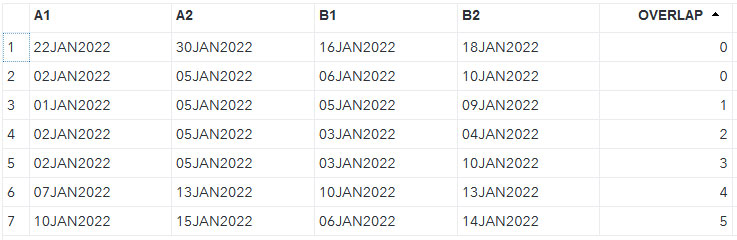Which of the presented here three methods do you like most? Which one would you prefer under different circumstances? Can you come up with yet another way of calculating date intervals overlaps? Do you have questions, concerns, comments? Please share with us below.

Calculating the overlap of date/time intervals was published on SAS Users.I am pretty sure you have never heard of the TRIMS function, and I would be genuinely surprised if you told me otherwise. This is because this function does not exist (at least at the time of this writing).

But don’t worry, the difference between "nonexistence" and "existence" is only a matter of time, and from now it is less than a blog away. Let me explain. Recently, I published two complementary blog posts:

While working on these pieces and researching “prior art” I stumbled upon a multipurpose function in the SAS FedSQL Language that alone does either one or both of these things – remove leading or/and trailing characters from SAS strings.

## FedSQL Language and Proc FedSQL

The FedSQL language is the SAS proprietary implementation of the ANSI SQL:1999 core standard. Expectedly, the FedSQL language is implemented in SAS by means of the FedSQL procedure (PROC FEDSQL). This procedure enables you to submit FedSQL language statements from a Base SAS session, and it is supported in both SAS 9.4 and SAS Viya.

Using the FEDSQL procedure, you can submit FedSQL language statements to SAS and third-party data sources that are accessed with SAS and SAS/ACCESS library engines. Or, if you have SAS Cloud Analytic Services (CAS) configured, you can submit FedSQL language statements to the CAS server.

## FedSQL TRIM function

FedSQL language has its own vast FedSQL Functions library with hundreds of functions many of which replicate SAS 9.4 Functions. Many, but not all. Deep inside this FedSQL functions library, there is a unique treasure modestly called TRIM Function which is quite different from the BASE SAS Language TRIM() function.

While SAS 9.4 BASE TRIM() function capabilities are quite limited - it removes just trailing blanks from a character string, the FedSQL TRIM() function is way much more powerful. This triple-action function can remove not just trailing blanks, but also leading blanks, as well as both, leading and trailing blanks. On top of it, it can remove not just blanks, but any characters (although one character at a time). See for yourself, this function has the following pretty self-explanatory syntax:

TRIM( [BOTH | LEADING | TRAILING] [trim-character] FROM column)

Here trim-character specifies one character (in single quotations marks) to remove from column. If trim-character is not specified, the function removes blanks.

While being called a function, it does not look like a regular SAS function where arguments are separated by commas.  It looks more like an SQL statement (which it understandably is – it is part of the FedSQL language). However, this function is available only in PROC FEDSQL; it’s not available in SAS DATA steps or other PROC steps. Still, it gives us pretty good idea of what such a universal function may look like.

## User-defined function TRIMS to remove leading or/and trailing characters in SAS strings

Let’s build such a function by means of the PROC FCMP for the outside the FedSQL usage (it is worth noticing that the FCMP procedure is not supported for FedSQL). To avoid confusion with the existing TRIM function we will call our new function TRIMS (with an ‘S’ at the end) which suits our purpose quite well denoting its plural purpose. First, we define what we are going to create.

### TRIMS Function

Removes leading characters, trailing characters, or both from a character string.

### Syntax

TRIMS(function-modifier, string, trim-list, trim-list-modifier)

### Required Arguments

• function-modifier is a case-insensitive character constant, variable, or expression that specifies one of three possible operations:
'L' or 'l' – removes leading characters.
'T' or 't' – removes trailing characters.
'B' or 'b' – removes both, leading and trailing characters.
• string is a case-sensitive character constant, variable, or expression that specifies the character string to be trimmed.
• trim-list is a case-sensitive character constant, variable, or expression that specifies character(s) to remove from the string.
• trim-list-modifier is a case-insensitive character constant variable, or expression that supplements the trim-list.
The valid values are those modifiers of the FINDC function that “add” groups of characters (e.g. 'a' or 'A', 'c' or 'C', 'd' or 'D', etc.) to the trim-list.

The following user-defined function implementation is based on the coding techniques described in the two previous posts,  and  that I mentioned above. Here goes.

```  libname funclib 'c:\projects\functions';   /* delete previous function definition during debugging */ options cmplib=funclib.userfuncs; proc fcmp outlib=funclib.userfuncs.package1; deletefunc trims; run;   /* new function defintion */ proc fcmp outlib=funclib.userfuncs.package1; function trims(f \$, str \$, clist \$, mod \$) \$32767; from = 1; last = length(str); if upcase(f) in ('L', 'B') then from = findc(str, clist, 'K'||mod); if from=0 then return(''); if upcase(f) in ('T', 'B') then last = findc(str, clist, 'K'||mod, -last); if last=0 then return(''); return(substr(str, from, last-from+1)); endfunc; run;```

### Code highlights

• In the function definition, we first assign initial values of the target substring positions as from=1 and last=length(str).
• Then for Leading or Both character removal, we calculate an adjusted value of from as a position of the first character in str that is not listed in clist and not defined by the mod
• If from=0 then we return blank and stop further calculations as this means that ALL characters are to be removed.
• Then for Trailing or Both character removal, we calculate an adjusted value of last as a position of the last character in str that is not listed in clist and not defined by the mod
• If last=0 then we return blank and stop further calculations as this means that ALL characters are to be removed.
• And finally, we return a substring of str starting at the from position and ending at the last position, that is with the length of last-from+1.

## TRIMS function usage

Let’s define SAS data set SOURCE as follows:

```data SOURCE; input X \$ 1-30; datalines; *00It's done*2*1**- *--*1****9*55 94*Clean record-*00 ;```

In the following DATA step, we will create three new variables with removed leading (variable XL), trailing (variable XT) and both - leading and trailing (variable XB) characters '*' and '-' as well as any digits:

```options cmplib=funclib.userfuncs; data TARGET; set SOURCE; length XB XL XT \$30; XB = trims('b', X, '*-', 'd'); XL = trims('L', X, '*-', 'd'); XT = trims('t', X, '*-', 'd'); run;```

In this code we use the TRIM function three times, each time with a different first argument to illustrate how this affects the outcome.

### Arguments usage highlights

• The first argument of the TRIMS function specifies whether we remove characters from both leading and trailing positions ('b'), from leading positions only ('L'), or from trailing positions only ('t'). This argument is case-insensitive. (I prefer using capital 'L' for clarity since lowercase 'l' looks like digit '1').
• The second argument specifies the name of the variable (X) that we are going to remove characters from (variable X is coming from the dataset SOURCE).
• The third argument '*-' specifies which character (or characters) to remove. In our example we are removing '*' and '-'. If you do not need to explicitly specify any character here, you still must supply a null value ('') since it is a required argument. In this case, the fourth argument (trim-list-modifier) will determine the set of characters to be removed.
• And finally, the fourth argument (case-insensitive) of the TRIMS function specifies the FINDC function modifier(s) to remove certain characters in bulk (in our example 'd' will remove all digits). If such modifier is not needed, you still must supply a null value ('') since all four arguments of the TRIMS function are positional and required.

Here is the output data table TARGRET showing the original string X and the resulting strings XB (Both leading and trailing characters removed), XL (Leading characters removed) and XT (Trailing characters removed) side by side: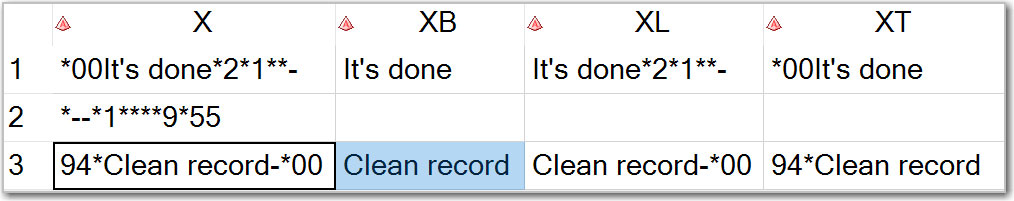## Conclusion

The new TRIMS function presented in this blog post goes ways further the ubiquitous LEFT and TRIM functions that remove the leading (LEFT) or trailing (TRIM) blanks. The TRIMS function handles ANY characters, not just blanks. It also expands the character deletion functionality of the powerful  FedSQL TRIM function beyond just removing any single leading and/or trailing character. The TRIMS function single-handedly removes any number of explicitly specified characters from either leading, trailing or both (leading and trailing) positions. Plus, it removes in bulk many implicitly specified characters. For example 'd' modifier removes all digits, 'du' modifier removes all digits ('d') and all uppercase letters ('u'), 'dup' modifier removes all digits ('d'), all uppercase letters ('u') and all punctuation marks ('p'); and so on as described by the FINDC function modifiers. The order in which modifier characters are listed does not matter.

Do you find this post useful? Please share your thoughts with us below.Just because you are using CAS actions doesn't mean you can forget about the powerful SAS DATA step. The dataStep.runCode CAS action is here!

Welcome back to my SAS Users blog series CAS Action! - a series on fundamentals. I've broken the series into logical, consumable parts. If you'd like to start by learning a little more about what CAS Actions are, please see CAS Actions and Action Sets - a brief intro. Or if you'd like to see other topics in the series, see the overview page.

In this example, I will use the CAS procedure to execute the dataStep.runCode CAS action. Be aware, instead of using the CAS procedure, I could execute the action with Python, R, or even a REST API with some slight changes to the syntax for the specific language.

## Why use the DATA Step?

It's pretty simple, the DATA step is a powerful way to process your data. It gives you full control of each row and column, ability to easily create multiple output tables, and provides a variety of statements to pretty much do anything you need.

In this example, I will use the DATA step to quickly create three CAS tables based on the value of a column.  Before we execute the DATA step, let's view the frequency values of the Origin column in the cars table. To do that, I'll use the simple.freq action.

```proc cas; simple.freq / table={name='cars', caslib='casuser'}, input='Origin'; quit;```

##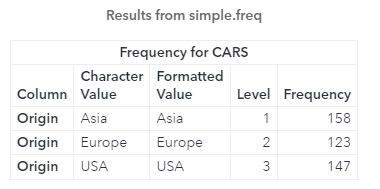The result of the freq action shows that the Origin column in the cars CAS table has three distinct values: Asia, Europe and USA. I can use that information to create three CAS tables based off these unique values using the SAS DATA step.

## Execute DATA Step in SAS Viya's CAS Server

One way to execute the DATA step directly in CAS is to use the runCode action with the code parameter. In the code parameter just specify the DATA step as a string. That's it!

In this example, I'll add the DATA step within a SOURCE block. The SOURCE block stores the code as variable. The DATA step code is stored in the variable originTables. This DATA step will create three CAS tables, one table for each unique value of the Origin column in the cars table.

```proc cas; source originTables; data casuser.Asia casuser.Europe casuser.USA; set casuser.cars; if Origin='Asia' then output casuser.Asia; else if Origin='Europe' then output casuser.Europe; else if Origin='USA' then output casuser.USA; run; endsource;   dataStep.runCode / code=originTables; quit;```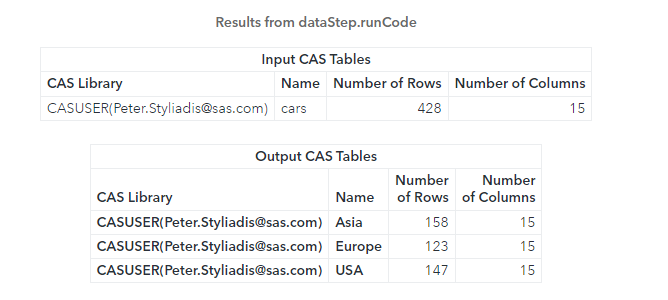The runCode action executes the DATA step in the distributed CAS environment and returns information about the input and output tables. Notice three CAS tables were created: Asia, Europe and USA.

## DATA Step in CAS has Limitations

Now, one thing to be aware of is not all functionality of the DATA step is available in CAS. If you are using the runCode action with an unsupported statement or function in CAS, you will receive an error. Let's look at an example using the first function, which gets the first letter of a string, and is not supported in CAS.

```proc cas; source originTables; data casuser.bad; set casuser.cars; NewCol=first(Model); run; endsource; dataStep.runCode / code=originTables; quit;```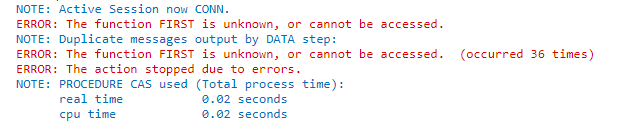The results of the runCode action return an error. The error occurs because the FIRST function is unknown or cannot be accessed. In situations like this you will need to find a CAS supported method to complete the task. (HINT: Here instead of the first function you can use the substr function).

For more information visit Restrictions and Supported Language Elements. Be sure to find the version of your SAS Viya environment.

## Summary

In SAS Viya, the runCode action provides an easy way to execute most of the traditional DATA step in CAS in any language, from the CAS Language (CASL), to Python, R, Lua, Java and more.

CAS-Action! Executing the SAS DATA Step in SAS Viya was published on SAS Users.Just because you are using CAS actions doesn't mean you can forget about the powerful SAS DATA step. The dataStep.runCode CAS action is here!

Welcome back to my SAS Users blog series CAS Action! - a series on fundamentals. I've broken the series into logical, consumable parts. If you'd like to start by learning a little more about what CAS Actions are, please see CAS Actions and Action Sets - a brief intro. Or if you'd like to see other topics in the series, see the overview page.

In this example, I will use the CAS procedure to execute the dataStep.runCode CAS action. Be aware, instead of using the CAS procedure, I could execute the action with Python, R, or even a REST API with some slight changes to the syntax for the specific language.

## Why use the DATA Step?

It's pretty simple, the DATA step is a powerful way to process your data. It gives you full control of each row and column, ability to easily create multiple output tables, and provides a variety of statements to pretty much do anything you need.

In this example, I will use the DATA step to quickly create three CAS tables based on the value of a column.  Before we execute the DATA step, let's view the frequency values of the Origin column in the cars table. To do that, I'll use the simple.freq action.

```proc cas; simple.freq / table={name='cars', caslib='casuser'}, input='Origin'; quit;```

##The result of the freq action shows that the Origin column in the cars CAS table has three distinct values: Asia, Europe and USA. I can use that information to create three CAS tables based off these unique values using the SAS DATA step.

## Execute DATA Step in SAS Viya's CAS Server

One way to execute the DATA step directly in CAS is to use the runCode action with the code parameter. In the code parameter just specify the DATA step as a string. That's it!

In this example, I'll add the DATA step within a SOURCE block. The SOURCE block stores the code as variable. The DATA step code is stored in the variable originTables. This DATA step will create three CAS tables, one table for each unique value of the Origin column in the cars table.

```proc cas; source originTables; data casuser.Asia casuser.Europe casuser.USA; set casuser.cars; if Origin='Asia' then output casuser.Asia; else if Origin='Europe' then output casuser.Europe; else if Origin='USA' then output casuser.USA; run; endsource;   dataStep.runCode / code=originTables; quit;```The runCode action executes the DATA step in the distributed CAS environment and returns information about the input and output tables. Notice three CAS tables were created: Asia, Europe and USA.

## DATA Step in CAS has Limitations

Now, one thing to be aware of is not all functionality of the DATA step is available in CAS. If you are using the runCode action with an unsupported statement or function in CAS, you will receive an error. Let's look at an example using the first function, which gets the first letter of a string, and is not supported in CAS.

```proc cas; source originTables; data casuser.bad; set casuser.cars; NewCol=first(Model); run; endsource; dataStep.runCode / code=originTables; quit;```The results of the runCode action return an error. The error occurs because the FIRST function is unknown or cannot be accessed. In situations like this you will need to find a CAS supported method to complete the task. (HINT: Here instead of the first function you can use the substr function).

For more information visit Restrictions and Supported Language Elements. Be sure to find the version of your SAS Viya environment.

## Summary

In SAS Viya, the runCode action provides an easy way to execute most of the traditional DATA step in CAS in any language, from the CAS Language (CASL), to Python, R, Lua, Java and more.

CAS-Action! Executing the SAS DATA Step in SAS Viya was published on SAS Users.In my previous post, we solved the task of removing specified leading characters from SAS strings. In this post, we tackle the complementary task of removing trailing characters.

While removing trailing blanks is well covered in SAS by the TRIM() and TRIMN() functions, removing non-blank trailing characters remains a bit of a mystery that can pop up during text string processing.

For example, you may need to clean up the following strings by removing all trailing x's from them:

012345x
012345xxx
012345xx

These extra characters can result from data entry errors, prior data manipulations, etc. No matter how you get them in, you want them out.

## How to remove any trailing characters

For instance, let’s remove all occurrences of the arbitrary trailing character '*'. The following diagram illustrates what we are going to achieve and how: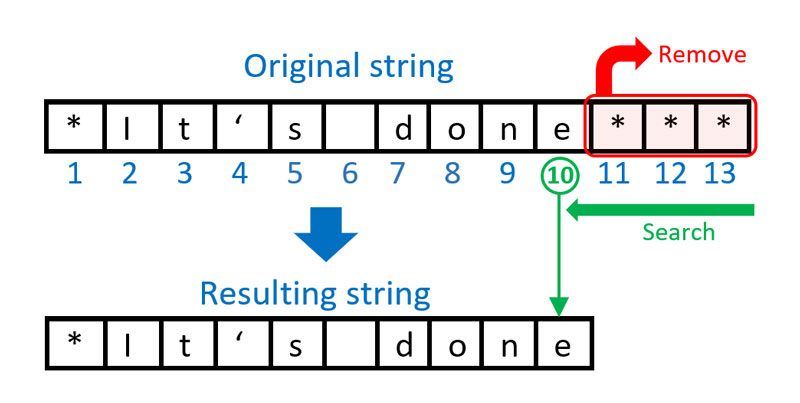In order to remove a specified character (in this example '*') from all trailing positions in a string, we need to search our string from right to left starting from the rightmost non-blank character position and find the position p of the first character in that string that is not equal to the specified character. Note, that despite our right-to-left direction of search the position p=10 is still enumerated from left-to-right. Then we can extract the substring starting from position 1 with the length equal to the found position p.

Unlike in our leading characters removal solution, out of two contenders for our search functionality, VERIFY and FINDC, the VERIFY function has to be dropped from the competition as it does not provide right-to-left search functionality. However, the FINDC function stays on track. Here is a possible solution using the FINDC() function.

#### Using FINDC() function with negative start-position

The FINDC(X, C, ’K’, -LENGTH(X)) function searches string X from right to left starting from the last non-blank character position determined by the optional start-position argument equal to LENGTH(X), and returns the position P of the first character that does not appear in C.

Here we use the K modifier that switches the default behavior of searching for any character that appears in C to searching for any character that does not appear in C.

The direction of search is defined by the minus sign in front of the start-position (a negative start-position argument translates into searching from right to left.)

Then we can apply the SUBSTR(X, 1, P) function that extracts a substring of X starting from position 1 with a length of P which is effectively a substring of the first P characters in X.

## Special considerations

Before we proceed to the code implementation of the outlined solution let’s consider the following edge case.

If our string X consists of all '*' characters and nothing else the FINDC() function will find no character (and therefore no position) that is not equal to '*'. In this case it will return 0. However, 0 is not a valid third argument value for the SUBSTR(X, 1, P) function. Valid values are 1 . . . through VLENGTH(X) – the length attribute of X. Having a 0 value for the third argument will trigger the automatic data step variable _ERROR_=1 and the following note generated in the SAS log:

NOTE: Invalid third argument to function SUBSTR at line ## column #.

Therefore, we need to handle this special case separately, conditionally using SUBSTR(X, 1, P) for P>0 and assigning blank ('') otherwise.

## Code implementation for removing trailing characters

Now we are ready to put everything together. First, let’s create a test data table:

```data TEST; input X \$ 1-20; datalines; *It's done*** ********* **01234*ABC** No trailing *'s ;```

Then we apply the logic described above. The following DATA step illustrates our coding solution for deleting trailing characters:

```data CLEAN (keep=X Y); set TEST; C = '*'; *<- trailing character(s) to be removed;   P = findc(X, C, 'K', -length(X)); if P then Y = substr(X, 1, P); else Y = '';   put _n_= / X= / P= / Y= /; run;```

The SAS log will show interim and final results by the DATA step iterations:

```_N_=1 X=*It's done*** P=10 Y=*It's done   _N_=2 X=********* P=0 Y=   _N_=3 X=**01234*ABC** P=11 Y=**01234*ABC   _N_=4 X=No trailing *'s P=15 Y=No trailing *'s```

Here is the output data table CLEAN showing the original and the resulting strings X and Y side by side: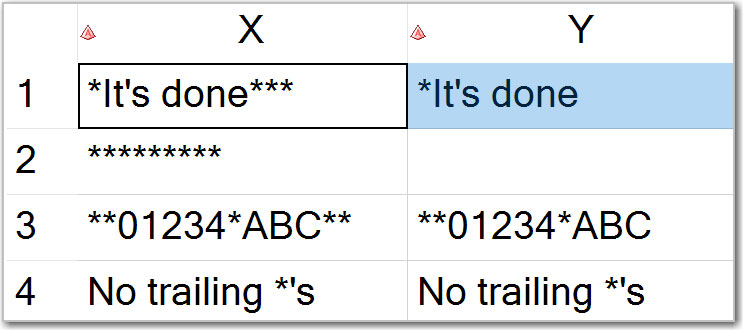## Conclusion

The solution presented in this blog post expands trailing character deletion functionality beyond solely blanks (which are handled by the TRIM and TRIMN functions). Moreover, using this coding technique, we can simultaneously remove a variety of trailing characters. For example, if we have a string X='012345xxx.%' and specify C = 'x.%' (the order of characters listed within the value of C does not matter), then all three characters 'x', '.', and '%' will be removed from all trailing positions of X. The resulting string will be Y='012345'.

In addition, numerous modifiers of the FINDC() function allow specifying many characters in bulk, without explicitly listing them one by one. For example, we may augment a list of characters being removed by adding the D modifier as in P = FINDC(X, C, 'KD', -LENGTH(X)) which will remove all trailing digits in addition to those characters specified in C. Similarly, we may throw in the U modifier as in P = FINDC(X, C, 'KDU', -LENGTH(X)) which adds all uppercase letters to the list of trailing characters to be removed. And so on.

Do you find this post useful? Do you have questions, concerns, comments? Please share with us below.

Removing trailing characters from SAS strings was published on SAS Users.As in many other programming languages, there is a very useful SAS function that removes leading blanks in character strings. It is the ubiquitous LEFT function.

The LEFT(x) function left-aligns a character string x, which effectively removes leading blanks.

However, in many SAS applications we need a similar but more versatile data cleansing functionality allowing for removal of other leading characters, not just blanks. For example, consider some bank account numbers that are stored as the following character strings:

123456789
0123456789
000123456789

These strings represent the same account number recorded with either no, one, or several leading zeros. One way of standardizing this data is by removing the leading 0's. And while we're at it, why don’t we address the leading character removal functionality for any leading characters, not just zeros.

## How to remove any leading characters

For example, let’s remove all occurrences of the arbitrary leading character '*'. The following diagram illustrates what we are going to achieve and how: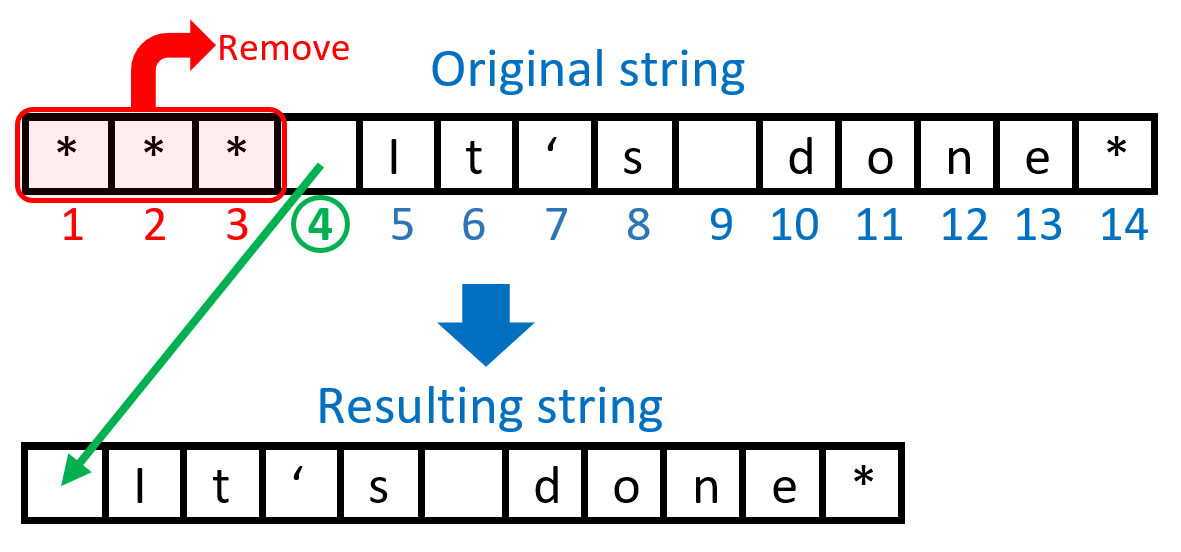In order to remove a specified character (in this example '*') from all leading positions in a string, we need to search our string from left to right and find the position of the first character in that string that is not equal to the specified character. In this case, it’s a blank character in position 4. Then we can extract a substring starting from that position till the end of the string.

I can see two possible solutions.

#### Solution 1: Using VERIFY() function

The VERIFY (X, C) function searches string X from left to right and returns the position P of the first character that does not appear in the value of C.

Then we can apply the SUBSTR(X,P) function that extracts a substring of X starting from position P till the end of the string X.

#### Solution 2: Using FINDC() function

The FINDC(X, C, ‘K’) function also searches string X from left to right and returns the position P of the first character that does not appear in C. (The modifier ‘K’ switches the default behavior of searching for any character that appears in C to searching for any character that does not appear in C.)

Then, as with the VERIFY() function, we can apply the SUBSTR(X,P) function that extracts a substring of X starting from position P till the end of the string X.

## Special considerations

So far so good, and everything will be just hunky-dory, right? Not really - unless we cover our bases by handling edge cases.

Have we thought of what would happen if our string X consisted of all '*' characters and nothing else? In this special case, both the verify() function and findc() function will find no position of the character that is not equal to '*' and thus return 0.

However, 0 is not a valid second argument value for the SUBSTR(X,P) function. Valid values are 1 . . . through length(X). Having a 0 value for the second argument will trigger the automatic data step variable _ERROR_=1 and the following note generated in the SAS log:

NOTE: Invalid second argument to function SUBSTR at line ## column #.

Therefore, we need to handle this special case separately, conditionally using SUBSTR(X,P) for P>0 and assigning blank ('') otherwise.

## Code implementation for removing leading characters

Let’s put everything together. First, we'll create a test data table:

```data TEST; input X \$ 1-20; datalines; *** It's done* ********* **01234*ABC** No leading *'s ;```

Then we apply the logic described above. The following DATA step illustrates our two implemented coding solutions for removing leading characters:

```data CLEAN (keep=X Y Z); set TEST; C = '*'; *<- leading character(s) to be removed;   P1 = verify(X,C); *<- Solution 1; if P1 then Y = substr(X, P1); else Y = '';   P2 = findc(X,C,'K'); *<- Solution 2; if P2 then Z = substr(X, P2); else Z = '';   put _n_= / X= / P1= / Y= / P2= / Z= /; run;```

Alternatively, we can replace the IF-THEN-ELSE construct with this IFC() function one-liner:

```data CLEAN (keep=X Y Z); set TEST; C='*'; *<- leading character(s) to be removed;   P1 = verify(X,C); *<- Solution 1; Y = ifc(P1, substr(X, P1), '');   P2 = findc(X,C,'K'); *<- Solution 2; Z = ifc(P2, substr(X, P2), '');   put _n_= / X= / P1= / Y= / P2= / Z= /; run;```

The SAS log will show interim and final results by the DATA step iterations:

```_N_=1 X=*** It's done* P1=4 Y=It's done* P2=4 Z=It's done*   _N_=2 X=********* P1=10 Y= P2=10 Z=   _N_=3 X=**01234*ABC** P1=3 Y=01234*ABC** P2=3 Z=01234*ABC**   _N_=4 X=No leading *'s P1=1 Y=No leading *'s P2=1 Z=No leading *'s```

Here is the output data table CLEAN showing the original string X, and resulting strings Y (solution 1) and Z (solution 2) side by side: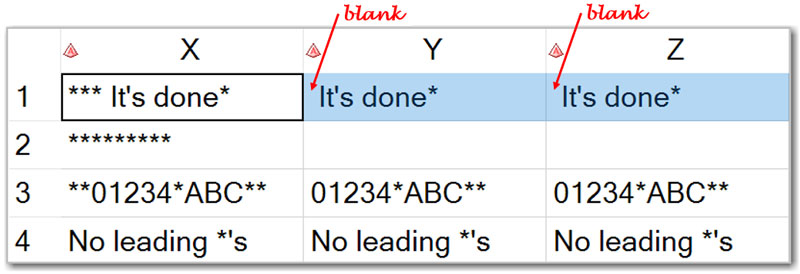As you can see, both solutions (1 & 2) produce identical results.

## Conclusion

Compared to the LEFT() function, the solution presented in this blog post not only expands leading character removal/cleansing functionality beyond the blank character exclusively. Using this coding technique we can simultaneously remove a variety of leading characters (including but not limited to blank). For example, if we have a string X=' 0.000 12345' and specify C = ' 0.' (the order of characters listed within the value of C does not matter), then all three characters ' ', '0', and '.' will be removed from all leading positions of X. The resulting string will be Y='12345'.

Do you find this post useful? Do you have questions, concerns, comments? Please share with us below.

Removing leading characters from SAS strings was published on SAS Users.Iterative loops are one of the most powerful and imperative features of any programming language, allowing blocks of code to be automatically executed repeatedly with some variations. In SAS we call them DO-loops because they are defined by the iterative DO statements. These statements come in three distinct forms:

• DO with index variable
• DO UNTIL
• DO WHILE

In this blog post we will focus on the versatile iterative DO loops with index variable pertaining to SAS DATA steps, as opposed to its modest IML’s DO loops subset.

## Iterative DO statement with index variable

The syntax of the DATA step’s iterative DO statement with index variable is remarkably simple yet powerful:

#### DO statement with index-variable

DO index-variable=specification-1 <, ...specification-n>;

...more SAS statements...

END;

It executes a block of code between the DO and END statements repeatedly, controlled by the value of an index variable. Given that angle brackets (< and >) denote “optional”, notice how index-variable requires at least one specification (specification-1) yet allows for multiple additional optional specifications (<, ...specification-n>) separated by commas.

Now, let’s look into the DO statement’s index-variable specifications.

#### Index-variable specification

Each specification denotes an expression, or a series of expressions as follows:

start-expression <TO stop-expression> <BY increment-expression> <WHILE (expression) | UNTIL (expression)>

Note that only start-expression is required here whereas <TO stop-expression>, <BY increment-expression>, and <WHILE (expression) or UNTIL (expression)> are optional.

Start-expression may be of either Numeric or Character type, while stop-expression and increment-expression may only be Numeric complementing Numeric start-expression.

Expressions in <WHILE (expression) | UNTIL (expression)> are Boolean Numeric expressions (numeric value other than 0 or missing is TRUE and a value of 0 or missing is FALSE).

## Other iterative DO statements

For comparison, here is a brief description of the other two forms of iterative DO statement:

• The DO UNTIL statement executes statements in a DO loop repetitively until a condition is true, checking the condition after each iteration of the DO loop. In other words, if the condition is true at the end of the current loop it will not iterate anymore, and processing continues with the next statement after END. Otherwise, it will iterate.
• The DO WHILE statement executes statements in a DO loop repetitively while a condition is true, checking the condition before each iteration of the DO loop. That is if the condition is true at the beginning of the current loop it will iterate, otherwise it will not, and processing continues with the next statement after the END.

## Looping over a list of index variable values/expressions

DO loops can iterate over a list of index variable values. For example, the following DO-loop will iterate its index variable values over a list of 7, 13, 5, 1 in the order they are specified:

```data A; do i=7, 13, 5, 1; put i=; output; end; run;```

This is not yet another form of iterative DO loop as it is fully covered by the iterative DO statement with index variable definition. In this case, the first value (7) is the required start expression of the required first specification, and all subsequent values (13, 5 and 1) are required start expressions of the additional optional specifications.

Similarly, the following example illustrates looping over a list of index variable character values:

```data A1; length j \$4; do j='a', 'bcd', 'efgh', 'xyz'; put j=; output; end; run;```

Since DO loop specifications denote expressions (values are just instances or subsets of expressions), we can expand our example to a list of actual expressions:

```data B; p = constant('pi'); do i=round(sin(p)), sin(p/2), sin(p/3); put i=; output; end; run;```

In this code DO-loop will iterate its index variable over a list of values defined by the following expressions: round(sin(p)), sin(p/2), sin(p/3).

## Infinite loops

Since <TO stop> is optional for the index-variable specification, the following code is perfectly syntactically correct:

```data C; do j=1 by 1; output; end; run;```

It will result in an infinite (endless) loop in which resulting data set will be growing indefinitely.

While unintentional infinite looping is considered to be a bug and programmers’ anathema, sometimes it may be used intentionally. For example, to find out what happens when data set size reaches the disk space capacity… Or instead of supplying a “big enough” hard-coded number (which is not a good programming practice) for the loop’s TO expression, we may want to define an infinite DO-loop and take care of its termination and exit inside the loop. For example, you can use IF exit-condition THEN LEAVE; or IF exit-condition THEN STOP; construct.

LEAVE statement immediately stops processing the current DO-loop and resumes with the next statement after its END.

STOP statement immediately stops execution of the current DATA step and SAS resumes processing statements after the end of the current DATA step.

The exit-condition may be unrelated to the index-variable and be based on some events occurrence. For instance, the following code will continue running syntactically “infinite” loop, but the IF-THEN-LEAVE statement will limit it to 200 seconds:

```data D; start = datetime(); do k=1 by 1; if datetime()-start gt 200 then leave; /* ... some processing ...*/ output; end; run;```

You can also create endless loop using DO UNTIL(0); or DO WHILE(1); statement, but again you would need to take care of its termination inside the loop.

## Changing “TO stop” within DO-loop will not affect the number of iterations

If you think you can break out of your DO loop prematurely by adjusting TO stop expression value from within the loop, you may want to run the following code snippet to prove to yourself it’s not going to happen:

```data E; n = 4; do i=1 to n; if i eq 2 then n = 2; put i=; output; end; run;```

This code will execute DO-loop 4 times despite that you change value of n from 4 to 2 within the loop.

According to the iterative DO statement documentation, any changes to stop made within the DO group do not affect the number of iterations. Instead, in order to stop iteration of DO-loop before index variable surpasses stop, change the value of index-variable so that it becomes equal to the value of stop, or use LEAVE statement to jump out of the loop. The following two examples will do just that:

```data F; do i=1 to 4; put i=; if i eq 2 then i = 4; output; end; run;   data G; do i=1 to 4; put i=; if i eq 2 then leave; output; end; run;```

## Know thy DO-loop specifications

Here is a little attention/comprehension test for you.

How many times will the following DO-loop iterate?

```data H; do i=1, 7, 3, 6, 2 until (i>3); put i=; output; end; run;```

If your answer is 2, you need to re-read the whole post from the beginning (I am only partly joking here).

You may easily find out the correct answer by running this code snippet in SAS. If you are surprised by the result, just take a closer look at the DO statement: there are 5 specifications for the index variable here (separated by commas) whereas UNTIL (expression) belongs to the last specification where i=2. Thus, UNTIL only applies to a single value of i=2 (not to any previous specifications of i =1,7,3,6); therefore, it has no effect as it is evaluated at the end of each iteration.

Now consider the following DO-loop definition:

```data Z; pi = constant('pi'); do x=3 while(x>pi), 10 to 1 by -pi*3, 20, 30 to 35 until(pi); put x=; output; end; run;```

I hope after reading this blog post you can easily identify the index variable list of values the DO-loop will iterate over. Feel free to share your solution and explanation in the comments section below.

Do you find this post useful? Do you have questions, other secrets, tips or tricks about the DO loop? Please share with us below.

Little known secrets of DO-loops with index variables was published on SAS Users.In many programming languages, there is a function named eval() that can be used to evaluate an expression and return the result at run time. For example, in Python, the eval() function parses the expression passed to it and runs a Python expression or code within the program. Even Python eval() supports more optional parameters, such as global/local dictionary for runtime context, but the goal for evaluation remains the same.

When an expression is stored as a character variable of an SAS observation, it suggests the running context is the SAS program execution phase, so the internal data structure like Input Buffer (IB), Program Data Vectors (PDV) and Descriptor Information of the output database are all accessible. Furthermore, all SAS system functions are also accessible in an expression, just like the raw SAS code exposed to SAS compiler and runtime. In the following SAS data set, what the user wants is to get the real value from the expression in variable C. It is different from the commonly used Calculated Column, which uses the same computing rule to expand a new variable for all observations. Here the column C has a different computing rule for each observation; the user's expected result is the column D.

```%let MVAR=2; data a; length a b 8 c \$ 255; a=3; b=4; c='a**2+b**2'; output; /* Arithmetic or Logical */ a=7; b=22; c='b/a * &MVAR'; output; /* SAS Macro Var*/ a=113; b=355; c='cos(b/a)'; output; /* Trigonometric */ a=0; b=1; c='cdf("NORMAL", 0, a, b)'; output; /* Probability */ run; proc print;run;```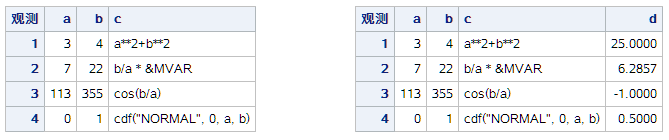Someone might want a solution that parses the expression in variable C, and then try to rebuild the abstract syntax tree and try to evaluate it from bottom to top. I can’t say this solution is totally wrong but it’s very complex and too hard to generate a general solution. If we have an SAS function eval() in DATA Step, then we can easily use the following code to achieve the goal. Unfortunately, SAS did not provide the eval() function.

```  data b; set a; d=eval(c); run;```

SAS provides Macro function %eval, %sysevalf to evaluate arithmetic and logical expressions using integer or floating-point arithmetic. SAS also provides function resolve() (not supported in the DATA Step that runs in CAS) to return the resolved value of the argument after it has been processed by the macro facility. Anyway, resolve() can’t access the values of macro variable assigned by symput() or symputn() at program execution phase. So, for the SAS code as below, it outputs c=150 and d=200. For simple arithmetic and logical expression, we can use the tranwrd() function to replace all variable names with real values, and then use resolve('%sysevalf('|| cats(expression_with_real_values) || ')') to get evaluated result, but it limited to only SAS functions supported by %eval() and %sysevalf() macro functions.

```%let a=100; %let b=50; data _null_; call symput("b", 200); c=resolve("%eval(&a+&b)"); put c=; d=symget("b"); put d=; run;```

Now let’s return to the key question we raised: How can we implement the eval() function in SAS Data Step? The best solution we found so far is to use the dynamic code generation mechanism in SAS, and then submit the code to SAS for parsing and execution. In this way, we retrieve string expression from a variable to a real expression in SAS code successfully. We don’t care what the valid SAS expression is, we totally convert it to the code snippets and submit it for execution. Syntax check, reference to PDV variables, internal functions, and even SAS Macro variables are all supported. Yes, it’s a smart and concise implementation for general purposes.

Due to each observation having a different computing rule, we need to use _N_ to control the observation mapping. So, for the existing dataset A, we can use the following code to achieve the goal. The key secret is to use CALL EXECUTE to generate SAS code dynamically and delay execution after the code is ready. In the output dataset B, we have a numeric column D with the value evaluated with the character expression from column C. You can see the output on the RIGHT part of the Figure 1.

```data _null_; set a end=last; if _n_=1 then call execute("data b; set a;"); call execute( "if _N_=" || compress(_N_) || " then d=" || trim(c) || "; "); if last then call execute("run;"); run; proc print; run;```

## Wrap up as a reusable SAS macro

We also can wrap up the above SAS code in a reusable SAS macro, so this logic can be used anywhere you want. The %evalvar macro has four arguments: ds= and var= are the input dataset and columns with expression to be evaluated. And outds= and outvar= are the output dataset and columns to store result. We also can specify same value for ds= and outds=, so the user just expands the existing dataset with an additional evaluated column.

```%macro EvalVar(ds=, var=, outds=, outvar=); data _null_; set &ds end=last; if _n_=1 then call execute("data &outds; set &ds;"); call execute( "if _n_=" || compress(_n_) || " then &outvar=" || &var || ";"); if last then call execute("run;"); run; %mend;   %EvalVar(ds=a, var=c, outds=b, outvar=d); proc print; run;```

By the way, I also had a temporary SAS code generation solution implemented via the %include SAS macro. The temporary SAS code will be deleted automatically when the SAS session is closed. The sample code is also attached here for your information.

```filename tmpfile temp; data _null_; file tmpfile; set a end=last; if _N_=1 then put "data b; set a;"; put "if _N_ =" _N_ "then d=" c ";"; if last then put "run;"; run; %include tmpfile; proc print; run;```

## Summary

In this article, we talk about how to evaluate SAS expressions in Data Step dynamically. The expression parsing and execution are totally handled by SAS at program execution phase. It avoids handling abstract syntax tree parsing and evaluation ourselves on it. We introduce two dynamic code generation implementations via call execute or %include. We also use _N_ to control observation mapping due to each observation has different computing rules. This implementation can reflect the beauty of simplicity in SAS before SAS provides system function eval() one day.

How to evaluate SAS expression in DATA Step dynamically was published on SAS Users.In this blog post we are going to tackle a data cleansing task of removing unwanted repeated characters in SAS character variables.

Character repetition can stem from various stages of data life cycle: from data collection, to data transmission, to data transformation. It can be accidental or intentional by design. It can be sporadic or consistent. In either case, it needs to be addressed by robust data cleansing processes to ensure adequate data quality that is imperative for the data usability.

## Character repetition examples

Example 1. Data entry, especially manual data entry, can be a high-risk factor for accidental character duplication. Have you ever pressed a key on your computer keyboard for a bit longer than intended, so it started automatically typing multiple characters???????????????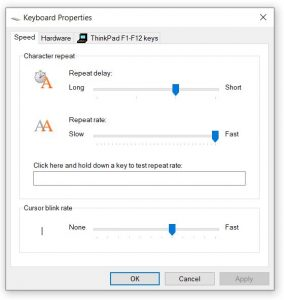Tip: You can adjust your Keyboard Properties to control “Repeat delay” and “Repeat rate” settings (on Windows computer, search for “Keyboard” and click on Keyboard in Control Panel).

Example 2. Recently, I had to deal with the data that contained multiple consecutive double quotation marks all over the character string values. Even though we don’t know the exact cause of it, still for each of these duplicated quotation marks occurrences we needed to replace them with a single quotation mark.

## Removing repeated blanks

There is a very useful Removing unwanted characters from text strings by Amadeus Software we developed a prototype using

 ```data D; c = ','; *<- character to un-duplicate; cc = c||c; *<- double character; string = 'Many,,,,,, commas,,,,, in,,, this,, sentence.,'; *<- source string; put 'BEFORE:' string=; *<- output initial string; do while (find(string,cc)); *<- loop through while there are doubles; string = tranwrd(string,cc,c); *<- replace double with a single character; end; put 'AFTER: ' string=; *<- output unduplicated string; run;```

This code will produce the following in the SAS log:

BEFORE:string=Many,,,,,, commas,,,,, in,,, this,, sentence.,
AFTER: string=Many, commas, in, this, sentence.,

which shows that this approach correctly un-duplicates the source string removing and replacing all repeated characters (commas in our example) with a single one.

## User-defined SAS function for removing any repeated characters

 ```libname funclib 'c:\projects\functions'; proc fcmp outlib=funclib.userfuncs.package1; function undupc(str \$, clist \$) \$; length x \$32767 c \$1 cc \$2; x = str; do i=1 to length(clist); c = char(clist,i); cc = c||c; do while (find(trim(x),cc)); x = tranwrd(x,cc,c); end; end; return (x); endfunc; run;```

Code highlights

• We introduce an interim variable x to which we will iteratively apply replacing double characters with a single one.
• We assign length attribute of this variable to be maximum allowable character length of 32767 bytes to accommodate any character length used in the calling program.
• Outer do-loop loops through the clist containing characters we want to unduplicate.
• Variable c is assigned a single character from clist, variable cc is assigned double of the cc value.
• Inner do-loop iterates through trimmed characters in x while doubles are found; using trim(x) is essential as it not only speeds up processing while searching through a shorter string (without trailing blanks), it also prevents from falling into an infinite loop in case clist contains blank character to unduplicate (cc equals to double blanks which are always going to be found among trailing blanks).

Let’s test our newly minted UNDUPC function on the following data:

```data SOURCE; infile datalines truncover; input str \$50.; datalines; """Repeated "double quotes"""" Repeated,,,,,commas,,,,,,,,,,, [[[""Mixed""]] characters,,, ;```
 ```options cmplib=funclib.userfuncs; data TARGET; set SOURCE; length new_str \$50; new_str = undupc(str, ' ,"]['); run;```

This code will remove and replace all repeated sequences of characters ' ',',', '"', ']', and '['. The order of these characters listed in the second argument doesn’t matter. Here is what we get: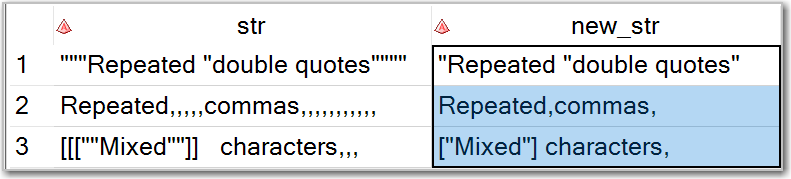As you can see, we get what we wanted including the functionality of the COMPBL function.

## User-defined CALL routine for removing any repeated characters

As much as I love user-defined functions, I have an issue with the above undupc user-defined function implementation. It has to do with how the PROC FCMP handles interim character variables length attribute assignment. It does not implicitly inherit their length attribute from another variable as SAS data step does. For example, if you run the following data step:

```data a; length x \$99; y = x; run;```

variable y will have the length attribute \$99 implicitly inherited from the x variable.

In PROC CFMP function, you can either assign the length attribute to a character variable explicitly with LENGTH or ATTRIB statement (as we did by using length x \$32767 ), or it will be set to \$33 if you use any other way of implicit assignment. (I leave it up to you guessing why 33 and why not any other number.) Since we wanted to accommodate SAS character strings of any length, we had to explicitly assign our interim variable x length attribute the maximum valid value of \$32767. This will inevitably take tall on the function performance as we will have to process longer strings.

However, we can avoid this issue by using CALL routine instead:

```libname funclib 'c:\projects\functions'; proc fcmp outlib=funclib.usercalls.package1; subroutine undupc(str \$, clist \$, x \$); outargs x; length c \$1 cc \$2; x = str; do i=1 to length(clist); c = char(clist,i); cc = c||c; do while (find(trim(x),cc)); x = tranwrd(x,cc,c); end; end; endsub; run;```
 ```options cmplib=funclib.usercalls; data TARGET; set SOURCE; length new_str \$50; call undupc(str, ' ,"][', new_str); run;```

And we will get the same results as with the UNDUPC function above.

## Store user-defined functions and subroutines separately

You can create and have both, user-defined function and call routine with the same name. However, to avoid confusion (and errors) do not store their definitions in the same data table (outlib= option of the PROC FCMP). If they are stored in the same data table, then when used in a DATA step, SAS will pull the latest definition by its name only and that may not be the entity you want.

## Performance benchmarking

To compare performances of the UNDUPC function vs UNDUPC subroutine we created a rather large data table (1 Million observations) with randomly generated strings (1000 characters long):

```libname SASDL 'C:\PROJECTS\TESTDATA';   data SASDL.TESTDATA (keep=str); length str \$1000; do i=1 to 1000000; str = ''; do j=1 to 1000; str = cats(str,byte(int(rank(' ')+38*rand('UNIFORM')))); end; output; end; run;```

Then we ran the following 2 data steps, one using the undupc() function, and the other using undupc() call routine:

```options cmplib=funclib.userfuncs;   data SASDL.TESTDATA_UNDUPC_FUNC; set SASDL.TESTDATA; length new_str \$1000; new_str = undupc(str, '#+'); run;   options cmplib=subrlib.usercalls;   data SASDL.TESTDATA_UNDUPC_CALL; set SASDL.TESTDATA; length new_str \$1000; call undupc(str, '#+', new_str); run;```

A quick SAS log inspection reveals that CALL UNDUPC works as much as 3 times faster than UNDUPC function (10 seconds vs. 30 seconds). The time savings may vary depending on your data composition and computing environment, but in any case, if you process high volumes of data you may consider using CALL routine over function. This is not a blanket statement, as it only pertains to this particular algorithm of eliminating character repetitions where we had to explicitly assign the highest possible length attribute value to the interim variable in the function, but not in the CALL routine.

When we reduced declared length of x from \$32767 to \$1000 within the user-defined function definition its performance became on par with the CALL routine.

## Additional Resources for SAS character strings processing